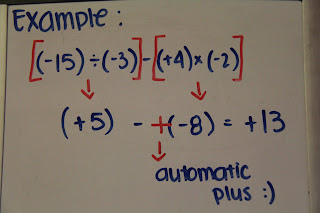## Tuesday, March 29, 2011

### Big book of integers chapeter

(+2)x(+3)= +6 (+2)x(-3= -6 (-2)x(+3)= +6 (-2)x(-3= +6

## Friday, March 25, 2011

### Ryan's Term 2 Reflection

I enjoyed the second term, because most of the units we did was very simple and easy for me. Although I didn’t do that well in quizzes/tests, volume and surface area was fun. It was easier for me to solve these kind of problems, because all I had to do was follow the formulas. These types of problems, were like an incomplete puzzle, where you knew what to do to complete them, and you would have to find each value of the formula, to subsitute them in. However, percents were a bit confusing for me. Other than the hundred grids, I did well. My only trouble with the hundred grids, were that if you needed to represent a number such as 0.06 percent, would you have to draw a “zoomed” version of a square, which was out of 100 squares? This confused me because there seemed like a much more simple way to represent that, without finding an equivalent fraction. Overall, I enjoyed the units we did this term, more than the units we did last term.

### Filimon's Volume Post

Pg 263 #5 v=h x pi x r2

r=4.1 11=h pi=3.14

r2 r x r = 16.81

Volume of this cylinder is 51.35.

#4 Pg 265

r2=10

h=23

pi=3.14

v=722.2

## Thursday, March 24, 2011

### Arween's Great Big Book Of Integers

Chapter 1 : Grade 7 Integer Review“When subtracting something that isn’t there use a zero pair”

Examples:-3 - (-7)=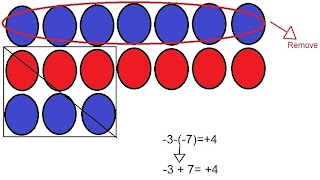-3 - 7 =3 - 7 =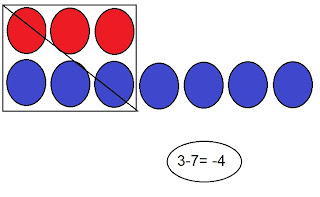3 + 7 =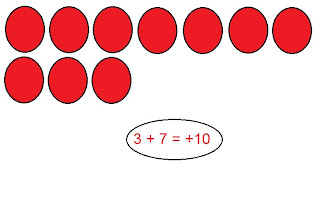-3 + 7 =Chapter 2 : Multiplying Integers
Sign rule: when you are multiplying integers and you have 0 or even numbers of negatives, the answer is positive. When you are multiplying integers and you have odd numbers of negatives, the answer is negative.

Examples:
(+2) x (+3)=(+2) x (-3)=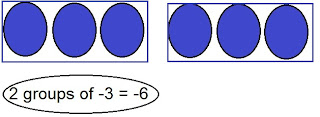(-2) x (+3)=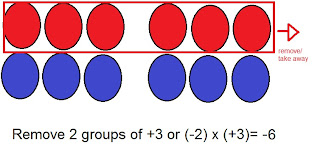(-2) x (-3)=Chapter 3 : Dividing Integers

Partitive Division is how many groups are there in a number.
6 ÷ 2 =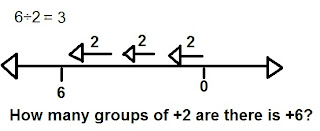Quotative division is how to share a number into groups
6 ÷ 2 =Sign rule:
Even: when numbers of negative integers are even the answer is positive
Odd: when numbers of negative integers are odd the answers is negative

6 ÷ 2 = 3 There are no negative integers so that means the quotient is positive.
-6 ÷ (-2) = 3 There are two (even) negative integers that means the quotient is positive.
(-6) ÷ 2 = -3 There is a negative integer (odd) that means the quotient is negative.
6÷(-2) = -3 There’s one negative integer that means the quotient is negative.

Chapter 4 : Order of Operations with Integers

(+5) x (-3) + (-6) ÷ (+3)
-15 + (-6) ÷ (+3)
-15 + -2
-17
When answering Order of Operations remember B.E.M.D.A.S
B = Brackets
E = Exponent
M = Multiplication
D = Division
S = Subtraction
1. First answer numbers with brackets Ex. ( ) or [ ]
2. Then Answer Multiplication and Division

### Roemer's Term 2 Reflection

This term we learned about percents. I found percents easy because I fund it easy and I think I will do good on the final exam. Also, we learned about Surface Area and Volume. Surface area and volume are our almost exactly alike. Only difference is on surface area, you want to find thetotal area of the shape, but in volume, you only have to find the "inside" area.I found this struggling in the beginning, but not anymore. With a little practise, I got much better. Math is usually one of my strongest subjects, but this term, I think I only got average. Next term, I will work 110% harder by completing homework, and getting better score test.

### Ishaka's Term 2 Reflection

This term we learned about surface area and volume. I think I did all right this term because when we were learning about surface area it was kind of hard but when we started to learn volume It was easy. I had great test scores It was probably because I started to pay attention in class. Learning volume was so easy that I didn’t struggle doing any of the work we did in class. Next term my goal is to do more work, and pay attention in class.

### Victoria's Great Big Book Of Integers Chapter 1

Grade 7 chapter 1 book of intergers : You can use number lines & integer chips. < - means red chip which has the symbol of a positive integer & > - means blue chip which has the symbol of a negative integer. For a zero pair looks like <> When subtracting something that isn't there use a zero pair. (+4)+(-4) =0 : thats how to equal a zero pair. Know becasue we are in grade 8 know more BRACKETS ! So it would look like 4 -4.

-6-(-4) =
-10+6 =
6-7+2 =
14-(-3) =
-3-(-7) =
* important to try to do
*-3-7 =
*3-7 =
*3+7 =
*-3+7=
For those questions use a numberline to complete .... Good Luck !

### Arween's 2nd Term Reflection

Term 2 Reflection

In Term 2 we’ve learned about Percents, Surface Areas, and Volumes. I did well on finding the surface area and finding the volume. The formulas of surface ares are easy to remember so as volumes. I struggled on percents. I had a hard time on changing fractions to percents. I keep messing up my calculating and mixing numbers to other numbers that’s in another column. But once I understand how to change fraction to percent I got better at it. I will do my work to understand the lecture more clearly to get a high grade on my quizzes.

### Filimon's Term 2 Reflection

This term with Mr. Harbeck was not a perfect term for me and my grade. I did not do my homework and he yelled at me. I had I lots of fun at Surface Area. I don’t remember what I got for the test and quiz’s, but I had lots of fun even if might of not got perfect grades. Surface Area was fun because I had to fall to get better and I did get better. I did not get AMAZING, but It was decent. I got a 60 something on math, I wanted to do better in math but I just find math boring. Learning area and integers is OK but I never saw the need to use it in the future, because I am probably not going to be a math related worker one day, but if I am will want to learn it in College.Even though I don't like math as much as much as Gym or L.A, bu I can be enjoyable. Thank You for reading my reflection.

### Paulo's Term 2 Reflection

This term was successful and bad for me. I did good in volume and surface area because I actually understand it. I did pretty bad in my quizzes/test except for the last test because I actually studied for it, when i started listening to Mr. Harbeck it was pretty simple to do. My goal for next term is to listen and study more. To achieve this goal what I'm going to do is listening and not get off task every time we do work and I'm going to use my time wisely at home to study and do more homework

## Wednesday, March 23, 2011

### Errol's Term 2 Reflection

In Term 2 I did good. I got an 87. I did really good in tests and quizzes. I found it easy to find surface area, volume of triangular prisms, cylinders and rectangular prisms, and percent.

I struggled on doing blogs posts and commenting. Even though I did better than last term, I still have a lot to do in term 3. This term I didn’t comment at all and had few blog post.

Next term(same as last term) I will try to comment on all blogs that I have to comment on and I will sign up for more blog posts.

This term I learned about surface area. Surface area is the sum of all faces of an object. For example: to find the surface area of a rectangular prism. Do L x W to one side, then multiply it by 2, and then do that to each side that’s different. Volume is the space on object occupies. For example: to find the volume of a rectangular prism, to the formula L x W x H. Percent means out of 100. For example: to find the percent from a decimal multiply by 100, so 0.64 x 100 = 64%.

There is a difference between volume and surface area. Surface area would be measuring the outside area of the object, while volume measures the inside area of an object. Both are similar because they’re both measuring an object.

### Jocelle's Term Two Reflection

This term, we learnt about percents, surface area, and volume. I understood all of the things it’s just I wasn’t really focusing on my work and for my recent quizzes, I did really bad because I wasn’t concentrating. This term was fun because I got to learn new things such as doing surface areas, even though I did bad, I tried my best to understand these kind of stuff. I think all of the people from our class enjoyed the things that we did because I don’t think any one of us failed, but some of us got higher marks or lower marks than their first term, but I promise that I will do better next term.

### Jocelyn's Term two reflection

This term we learned about percents. Percents were alright they weren't the greatest but I could at least understand what I have to do in order to do good on percents in the final exam. We also learned about surface area and volume. Surface area and volume are sort of similar but surface area you want to find the area of the whole shape, where as volume you only have to find the "inside" area. I struggled with both of these when we first started these units because it was sort of new to me but now I get the hang of it and I understand it better. Math was definitely not the best subject this term but I'm going to work twice as hard so that I'm able to complete assignments and get good test marks.

### Jocelle’s Great Big Book of Integers

Chapter 1 : Grade 7 Integer Review

The Sign Rule :

Chapter 3 : Dividing Integers

Example:

The sign rule for division is the same as the sign rule for multiplication, addition, and subtraction.

6 ÷ 2 = 3 There are no negative signs in the question, therefore the answer it positive.

-6 ÷ (-2) = 3 There is an even number of negative signs in the question, so the answer is positive.

(-6) ÷ 2 = -3 There is an odd number (one) of negative signs in the question, therefore the answer is negative.

6 ÷ (-2) = -3 There is one negative sign in the question, therefore the answer is negative.

Chapter 4 : Order of Operations with Integers

Use B.E.D.M.A.S. to solve equations except "E"